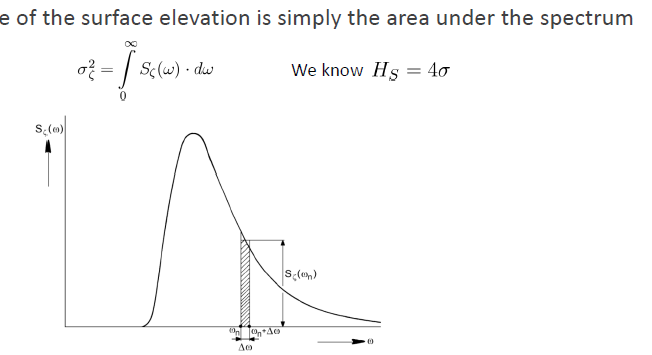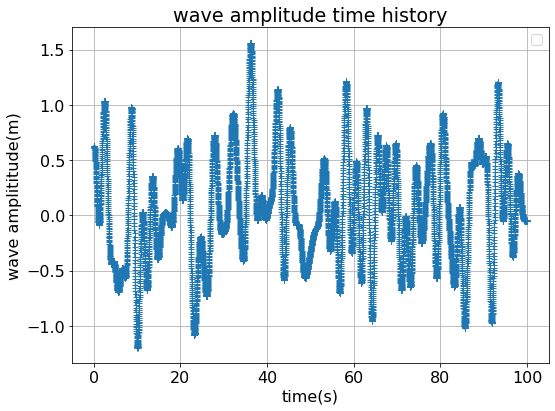# White-noise wave spectrum in OpenFast

Dear jason,

I am using the white-noise wave spectrum to generate irregular waves to explore the motion of the OC3 system in waves, to verify my calculations, I used openfast simulation results as a reference. When using the openfast, I set the significant wave height to 2m and got some results, the wave time curve at point (0,0) is as follows:

The significant wave height is calculated as follows:So, when Hs = 2m, the area under the spectrum should be 0.25, my frequency range is 0-3rad/s, so the value of S(w) should always be 0.25/3 m^2*s/rad. The wave spectrum is as folows:

Later, I use this white noise spectrum to generate irregular waves, however, compared to the OpenFast’s results, my wave amplitude is smallMay I ask if my understanding of this issue is wrong? I would appreciate it if you could give me some advice.

Best regards
Lin.Yang

Dear @Lin.Yang2,

I believe the issue with your math is that you use Hs = 4*sigma, which is only valid for a narrow-banded sea state, rather than the classic definition of Hs being the mean of the 1/3 highest waves.

When `WaveMod` is set to 3 in HydroDyn (white noise), HydroDyn computes the 1-sided wave spectrum as:

`WaveS1Sdd = WaveHs*WaveHs / ( 8*( WvHiCOff - WvLowCOff ) )`

which in your case would be:

WaveS1Sdd = 22 / ( 83 ) = 0.16666 m^2/(rad/s)

Best regards,

Dear jason,